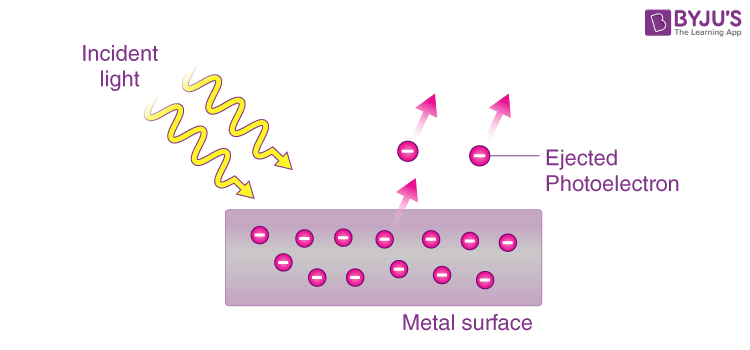# Particle Nature of Light

The emission of free electrons from a metal surface when light is shone on it, is called photoemission or the photoelectric effect. This effect led to the conclusion that light is made up of packets or quantum of energy. Now the question was whether the light quantum theory was indicative of the particle nature of light. Einstein had already associated the light quantum with momentum. This strongly supported the particle nature of light and these particles were named photons. Thus, the wave-particle duality of light came into the picture.### Photons:

Some points to be kept in mind are:
• A photon is an elementary particle. It is a quantum of light.
• The energy of a photon is given by
$$\begin{array}{l}E = h\nu\end{array}$$
Its momentum is
$$\begin{array}{l}p = \frac{h\nu }{c}\end{array}$$
and speed is c, which is the speed of light.
• Irrespective of the intensity of radiation, every photon of a frequency v has the same momentum
$$\begin{array}{l}p = \frac{h\nu }{c}\end{array}$$
and energy
$$\begin{array}{l}E = h\nu\end{array}$$
• The increase in the intensity of light only increases the number of photons crossing an area per unit time. It does not affect the energy of the radiation.
• A photon remains unaffected by electric and magnetic fields. It is electrically neutral.
• A photon has a zero mass, i.e. it is massless.
• It is a stable particle.
• Photons can be created or destroyed when radiation is emitted or absorbed.
• The total energy and momentum are conserved during a photon-electron collision.
• A photon cannot decay on its own.
• The energy of a photon can be transferred during an interaction with other particles.
• A photon is a spin-1 particle, unlike electrons which are ½ spin. It’s spin axis is parallel to the direction of travel. It is this property of photons that supports the polarization of light.

Stay tuned with BYJU’S for more such interesting articles. Also, register to “BYJU’S – The Learning App” for loads of interactive, engaging Physics-related videos and an unlimited academic assist.

## Frequently Asked Questions – FAQs

Q1

### What is light?

Light is the electromagnetic radiation within the section of the electromagnetic spectrum that is visible to the human eye.
Q2

### How is light produced?

Light is made up of elementary particles called photons. Photons are tiny energy packets. When a material’s atoms get heated or excited, photons are generated from the intense motion of atoms. The hotter the body, the more photons are created.
Q3

### What is the photoelectric effect?

The emission of free electrons from a metal surface when light is incident on it, is called the photoelectric effect.
Q4

### What is the mass of a photon?

A photon has a zero mass, i.e. it is massless.
Q5

### What is the effect of the magnetic field on photons?

A photon remains unaffected by electric and magnetic fields. It is electrically neutral.
Test your knowledge on Particle Nature Light Photons